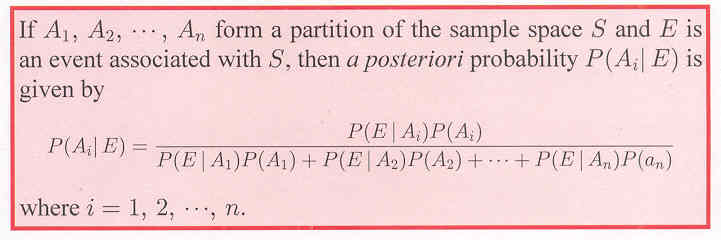# Section 13.5: Bayes’ Theorem

## 13.5 Outline

1. Probabilities with a partition of a sample space
1. partition of a set
2. probability of a partitioned event
2. A posteriori probabilities
1. a priori probabilities
2. a posteriori probabilities
3. Bayes’ theorem

## 13.5 Essential Ideas

Partition of a set:  A set is said to be partitioned if is divided into subsets satisfying the following two conditions.

1.  If and be any two subsets, then That is, the members of the subsets are pairwise disjoint.
2. The union of all the subsets is ; that is, there are no elements of that are not included in
one of the subsets.

Probability of a partitioned event: If A1, A2, …, An form a partition of a sample space E is any event, then

P(E) = P(E|A1)P(A1)
+ P(E|A2)P(A2)
+ … + P(E|An)P(An)

Bayes’ theorem: